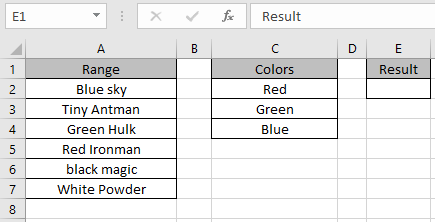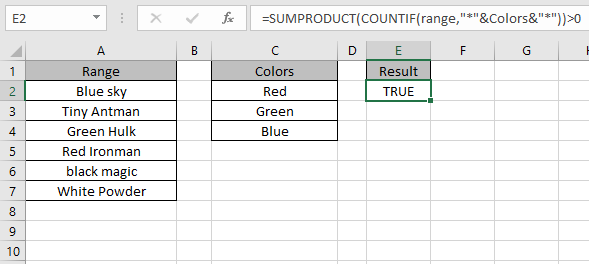# Excel If Range of Cells Contains Specific Text

In this article, we will learn how to know if a range contains specific text or not.

For instance, you have a large list of data and you need to find the presence of substrings in a range using excel functions. For this article we will need to use 2 functions:

1. SUMPRODUCT Function
2. COUNTIF function with wildcard

SUMPRODUCT function is a mathematical function in Excel. It operates on multiple ranges. It multiplies the corresponding arrays and then adds them.

The COUNTIF function of excel just counts the number of cells with a specific condition in a given range.

A wildcard is a special character that lets you perform indefinite matching on text in your Excel formulas.

There are three wildcard characters in Excel

1. Question mark (?)
2. Asterisk (*)
3. Tilde (~)

Generic formula:

= SUMPRODUCT( COUNTIF ( range , "*" &substrings& "*" ) ) > 0

Explanation:

• Here we need to apply the asterisk ( * ) with each substring. Asterisk ( * ) matches any number of characters when used.
•  Then COUNTIF function takes argument of range & substrings with wildcard and counts the number of matched cells.
• SUMPRODUCT function takes the numbers returned by COUNTIF function and gets their SUM.
• The formula checks the outcome number with >0 and returns TRUE if the statement stands TRUE or else the formula returns FALSE.

Example:

Here we have data in range A2 : A7. we need to find the presence of Colors in Range using this formula.Use the Formula:

= SUMPRODUCT ( COUNTIF ( range , "*" &Colors& "*" ) ) > 0

Explanation:

• Here the asterisk ( * ) is applied with each substring color. Asterisk ( * ) matches any number of characters when used.
•  Then COUNTIF function takes argument of range & color with wildcard and counts the number of matched cells which comes out to be.

= SUMPRODUCT ( COUNTIF ( range , { *Red* ; "Green" ; "Blue" ))>0

• SUMPRODUCT function takes the numbers returned by COUNTIF function and gets their SUM which comes out here as.

=SUMPRODUCT ( { 1 ; 1 ; 1 } ) > 0

• The formula checks the outcome ( 3 ) number with >0 and returns TRUE.

Here the range is named range for A2 : A7 & Colors named for the substrings in C2 : C4.As you can see in the above snapshot, the formula returns TRUE stats that the substring colors are found in the Range values.

Notes:

1. The formula returns #VALUE! error if the argument to the function is numeric.
2. The formula returns the wrong result if the formula syntax is not correct.

Hope this article about whether Range contains value using wildcards in Excel is explanatory. Find more articles on SUMPRODUCT functions here. Please share your query below in the comment box. We will assist you.

Related Articles

How to use the SUMPRODUCT function in excel

How to use the COUNTIF function in excel

How to use the WILDCARDS function in excel

How to Remove Text in Excel Starting From a Position

Validation of text entries

Create drop down list in excel with colour

Remove leading and trailing spaces from text in Excel

Popular Articles

Edit a dropdown list

Absolute reference in Excel

If with conditional formatting

If with wildcards

Vlookup by date

Join first and last name in excel

Count cells which match either A or B

Convert Inches To Feet and Inches in Excel 2016

50 Excel Shortcut to Increase Your Productivity

Terms and Conditions of use

The applications/code on this site are distributed as is and without warranties or liability. In no event shall the owner of the copyrights, or the authors of the applications/code be liable for any loss of profit, any problems or any damage resulting from the use or evaluation of the applications/code.